Courses
Courses for Kids
Free study material
Offline Centres
More

# NCERT Solutions for Class 11 Maths Chapter 6 - ExerciseLast updated date: 22nd Nov 2023
Total views: 529.8k
Views today: 11.29k

## NCERT Solutions for Class 11 Maths Chapter 6 Linear Inequalities (Ex 6.2) Exercise 6.2

NCERT Solutions for Class 11 Maths Chapter 6 exercise 6.2 are now available on the Vedantu website. The solutions have been prepared by our experts at Vedantu. These solutions are prepared in such a way that it would be easy to understand the chapter better and even get to know the tricks to solve the questions. The mathematics subject of standard 11 is one of the important subjects. So download Class 11 Maths Chapter 6 exercise 6.2 and get good scores in your examinations.

## Access NCERT Solutions for Class 11 Maths Chapter 6 – Linear Inequalities

Exercise 6.2

1. Solve the given inequality graphically in two-dimensional plane:

$x+y<5$

Ans: The graphical representation of the given plane $x+y<5$ is given below.

The plane divides the $\text{x-y}$ plane into two planes $\text{I}$ and $\text{II}$.

Let us select a point which lies in any of the planes to check if the point satisfies the inequality or not.

Let us take $\left( 0,0 \right)$, we get

$0+0<5$

$0<5$

Therefore we can say that the point satisfies the inequality.

Thus, we can say that the half plane $\text{II}$ does not satisfy the given inequality.

So, the graph is represented as below: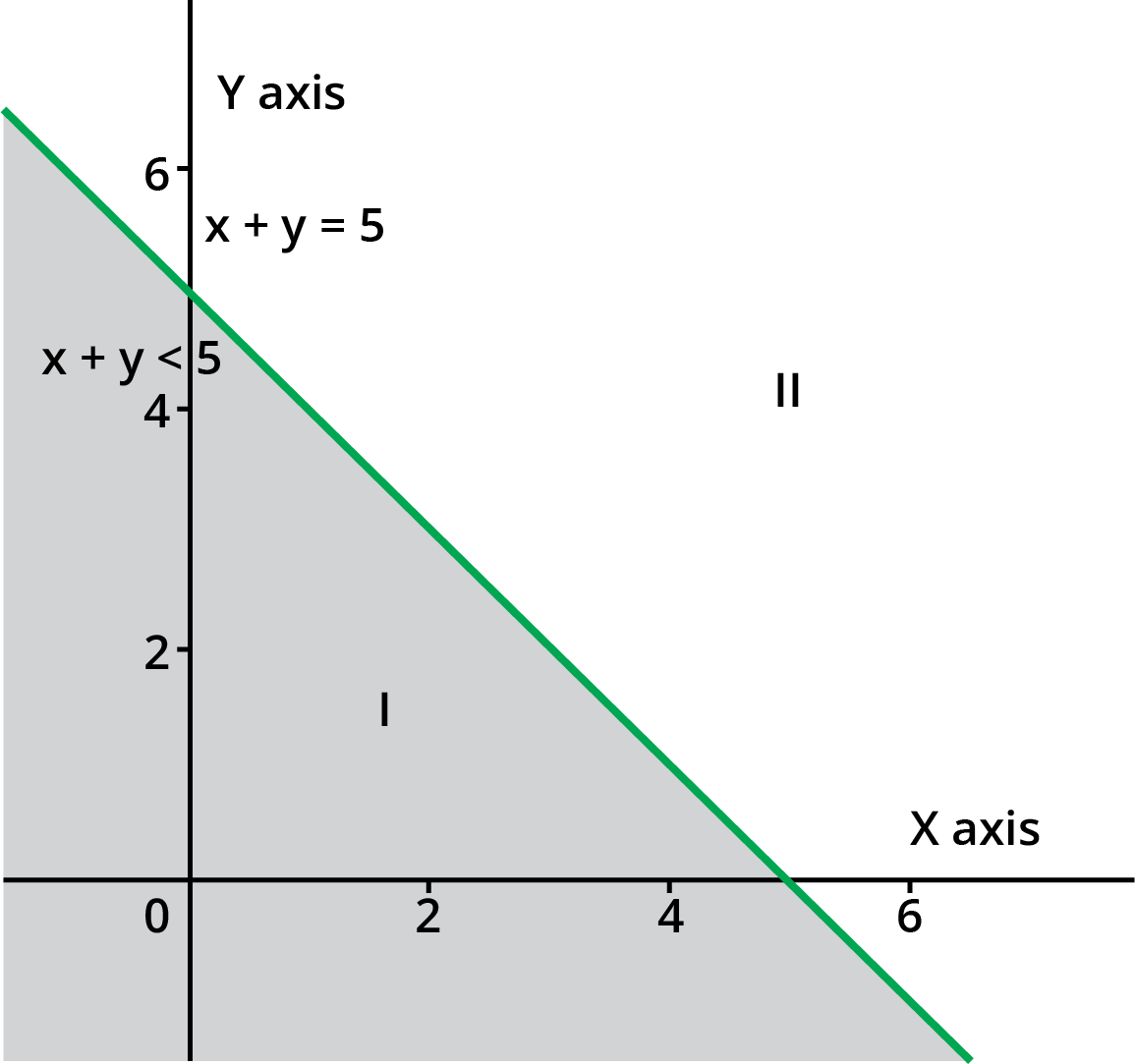2. Solve the given inequality graphically in two-dimensional plane:

$2x+y\geq6$

Ans: The graphical representation of the given plane $2x+y\geq6$ is given below.

The plane divides the $\text{x-y}$ plane into two planes $\text{I}$ and $\text{II}$.

Let us select a point which lies in any of the planes to check if the point satisfies the inequality or not.

Let us take $\left( 4,7 \right)$, we get

$2\left( 4 \right)+7\geq6$

$8+7\geq6$

$15>6$

Therefore we can say that the point satisfies the inequality.

It is also clear that any point on line also satisfies the given inequality.

Thus, we can say that the half plane $\text{II}$ does not satisfy the given inequality.

So, the graph is represented as below: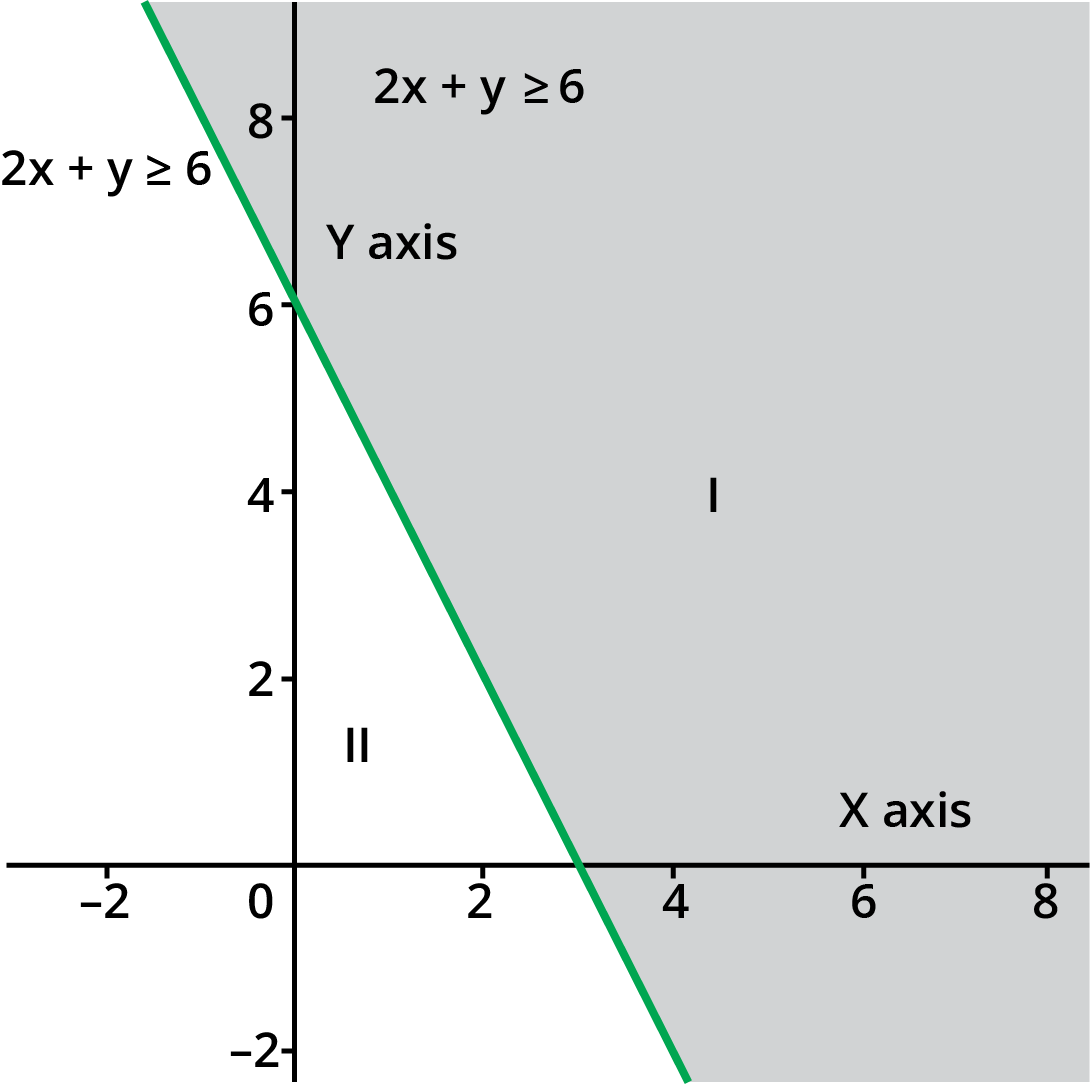3. Solve the given inequality graphically in two-dimensional plane: $3x+4y\leq12$

Ans: The graphical representation of the given plane $3x+4y\leq12$ is given below.

The plane divides the $\text{x-y}$ plane into two planes $\text{I}$ and $\text{II}$.

Let us select a point which lies in any of the planes to check if the point satisfies the inequality or not.

Let us take $\left( 0,0 \right)$, we get

$3\left( 0 \right)+4\left( 0 \right)\leq12$

$0\leq{{12}_{_{{}}}}$

Therefore we can say that the point satisfies the inequality.

It is also clear that any point on line also satisfies the given inequality.

Thus, we can say that the half plane $\text{II}$ does not satisfy the given inequality.

So, the graph is represented as below: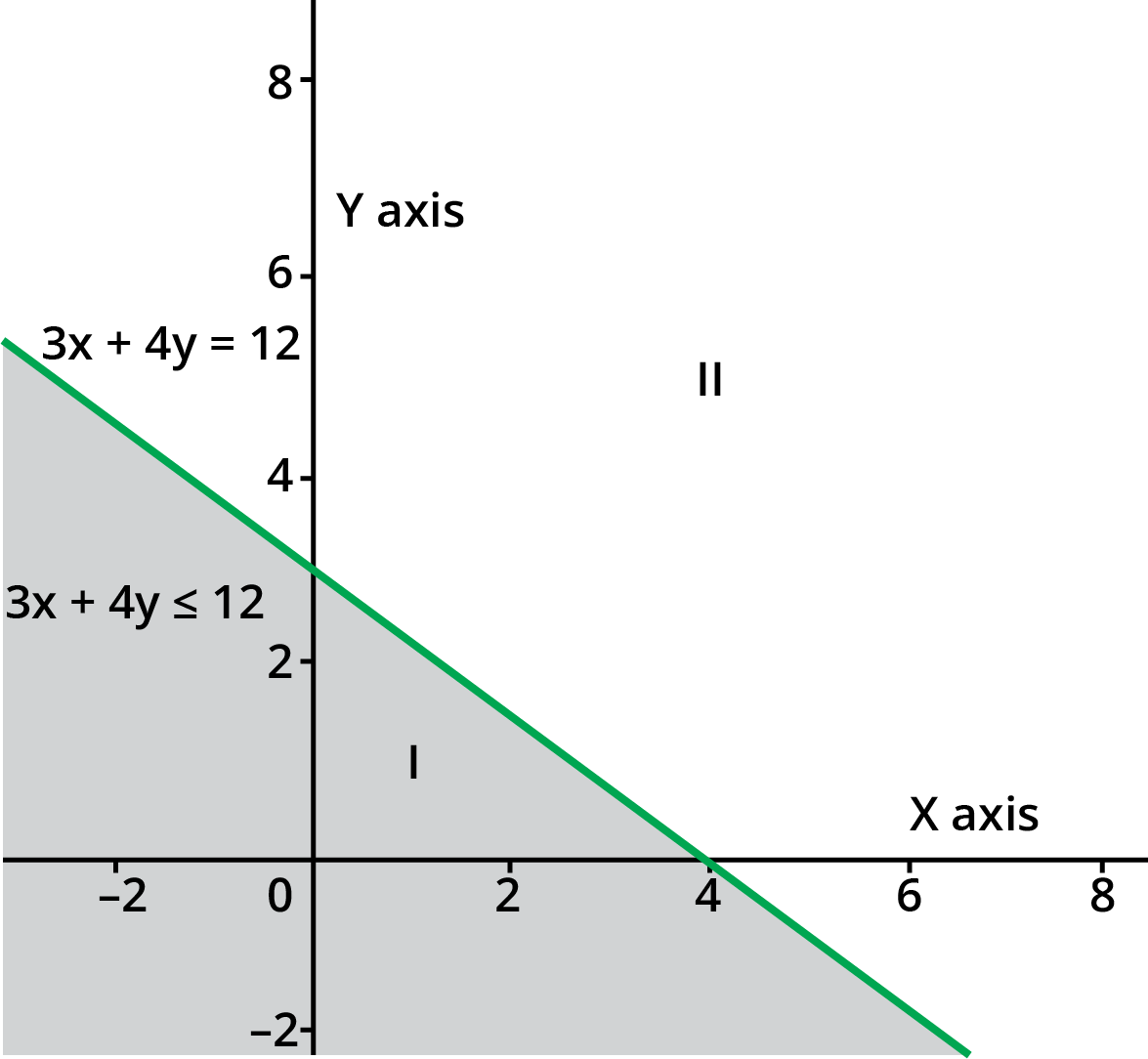4. Solve the given inequality graphically in two-dimensional plane: $y+8\geq2x$

Ans:

The graphical representation of the given plane $y+8\geq2x$ is given below.

The plane divides the $\text{x-y}$ plane into two planes $\text{I}$ and $\text{II}$.

Let us select a point which lies in any of the planes to check if the point satisfies the inequality or not.

Let us take $\left( 0,0 \right)$, we get

$0+8\geq2\left( 0 \right)$

$8\geq0$

Therefore we can say that the point satisfies the inequality.

It is also clear that any point on line also satisfies the given inequality.

Thus, we can say that the half plane $\text{II}$ does not satisfy the given inequality.

So, the graph is represented as below: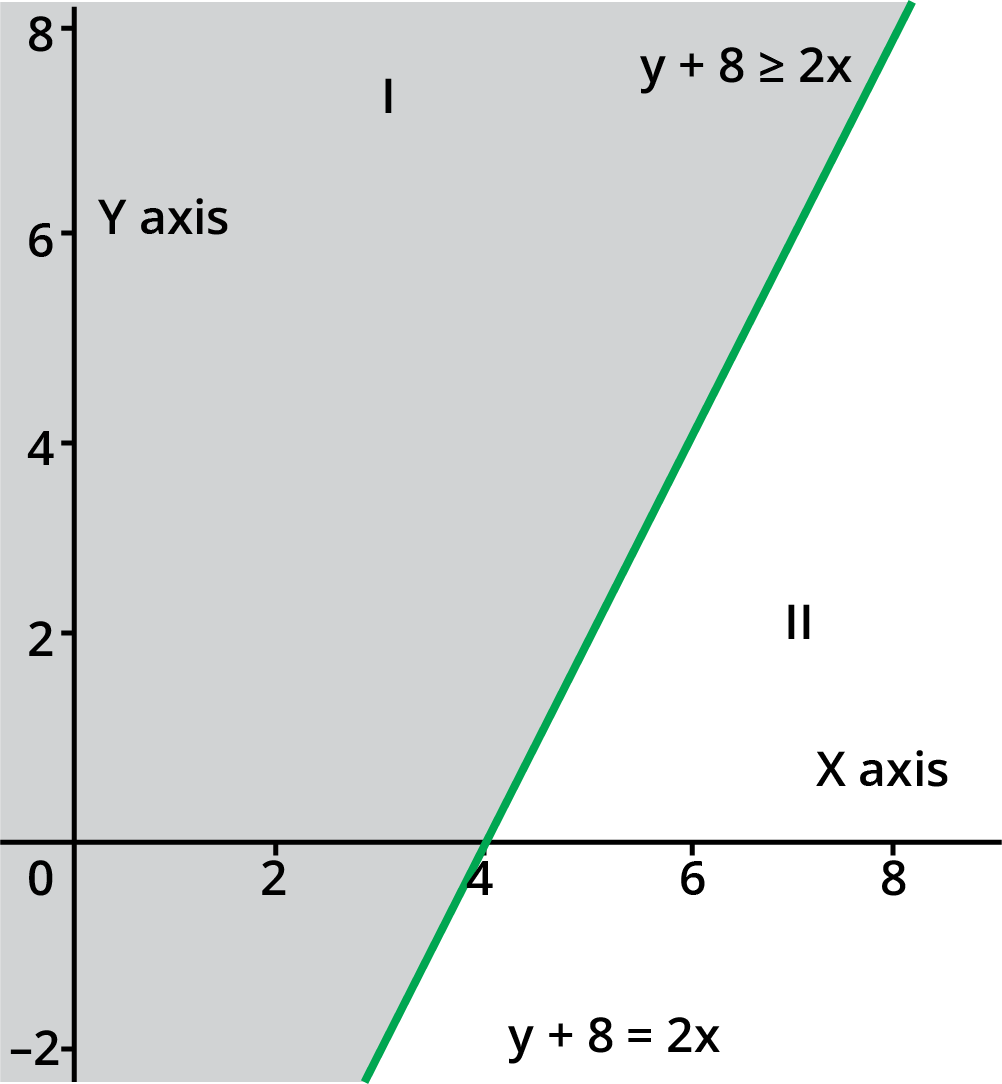5. Solve the given inequality graphically in two-dimensional plane:

$x-y\leq2$

Ans: The graphical representation of the given plane $x-y\leq2$ is given below.

The plane divides the $\text{x-y}$ plane into two planes $\text{I}$ and $\text{II}$.

Let us select a point which lies in any of the planes to check if the point satisfies the inequality or not.

Let us take $\left( 0,0 \right)$, we get

$0-0\leq2$

$0\leq2$

Therefore we can say that the point satisfies the inequality.

It is also clear that any point on line also satisfies the given inequality.

Thus, we can say that the half plane $\text{II}$ does not satisfy the given inequality.

So, the graph is represented as below: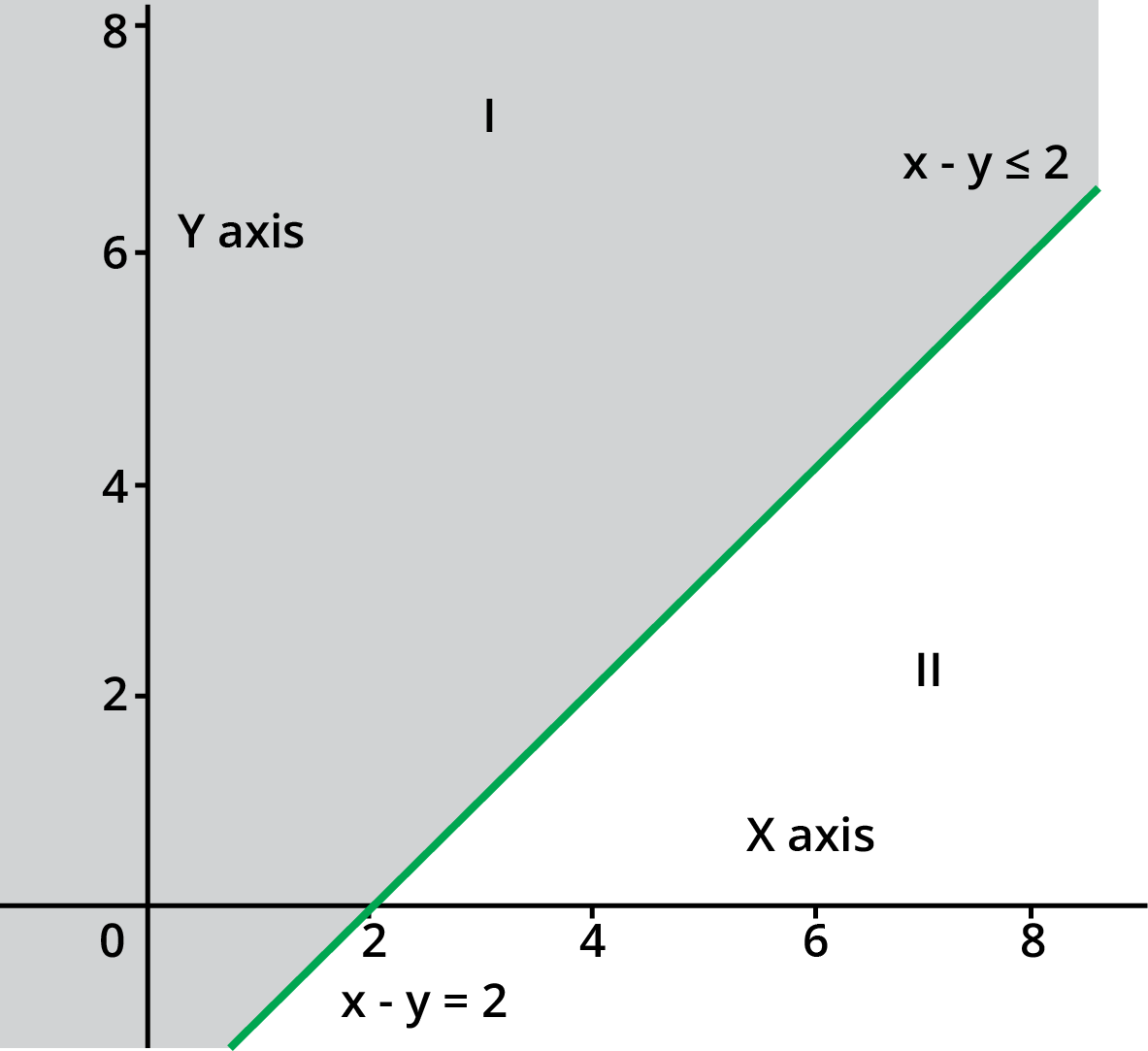6. Solve the given inequality graphically in two-dimensional plane: $\mathrm{2x-3y>6}$

Ans: The graphical representation of the given plane $2\text{x-3y>6}$ is given below.

The plane divides the $\text{x-y}$ plane into two planes $\text{I}$ and $\text{II}$.

Let us select a point which lies in any of the planes to check if the point satisfies the inequality or not.

Let us take $\left( 4,-1 \right)$, we get

$2\left( 4 \right)-3\left( -1 \right)>6$

$8+3>6$

$11>6$

Therefore we can say that the point satisfies the inequality.

Thus, we can say that the half plane $\text{II}$ does not satisfy the given inequality.

So, the graph is represented as below: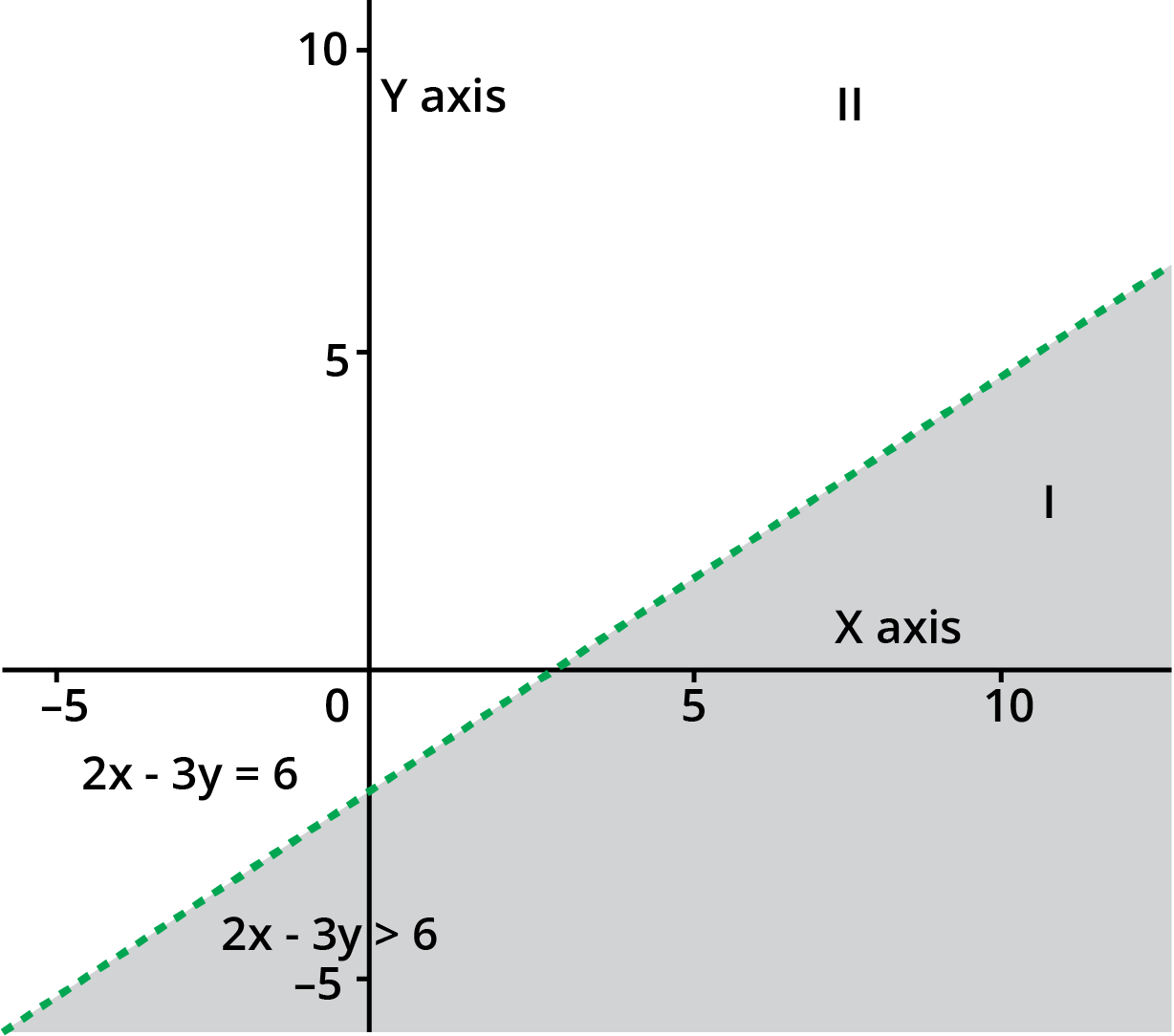7. Solve the given inequality graphically in two-dimensional plane: $\mathrm{-3x+2y\geq-6}$

Ans: The graphical representation of the given plane $\mathrm{-3x+2y\geq-6}$

is given below.

The plane divides the $\text{x-y}$ plane into two planes $\text{I}$ and $\text{II}$.

Let us select a point which lies in any of the planes to check if the point satisfies the inequality or not.

Let us take $\left( 0,0 \right)$, we get

$-3\left( 0 \right)+2\left( 0 \right)\geq-6$

$0\geq-6$

Therefore we can say that the point satisfies the inequality.

It is also clear that any point on line also satisfies the given inequality.

Thus, we can say that the half plane $\text{II}$ does not satisfy the given inequality.

So, the graph is represented as below: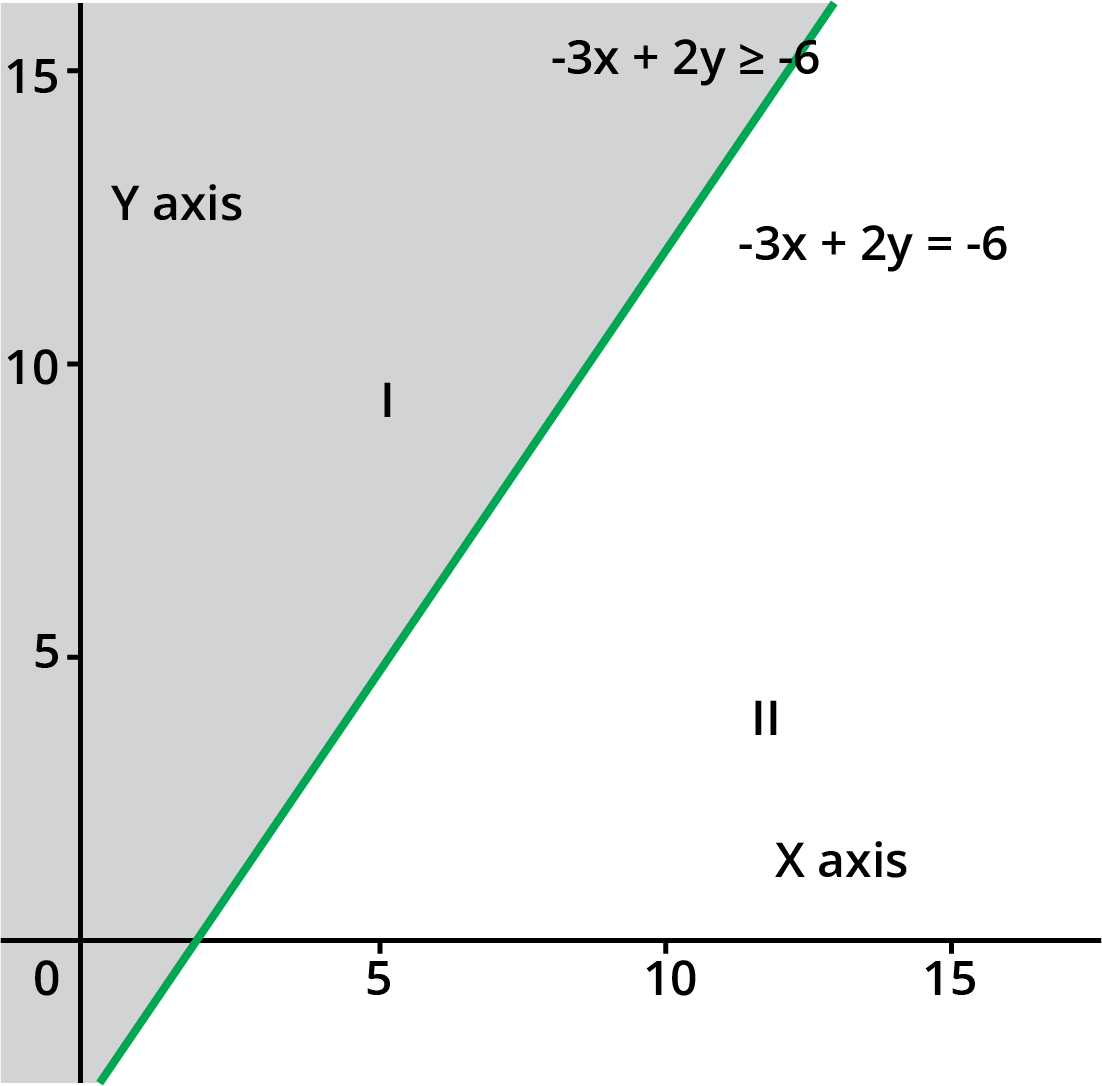8. Solve the given inequality graphically in two-dimensional plane: $\mathrm{3y-5x<30}$

Ans: The graphical representation of the given plane $3y-5x<30$ is given below.

The plane divides the $\text{x-y}$ plane into two planes $\text{I}$ and $\text{II}$.

Let us select a point which lies in any of the planes to check if the point satisfies the inequality or not.

Let us take $\left( 0,0 \right)$, we get

$3\left( 0 \right)-5\left( 0 \right)<30$

$0<30$

Therefore we can say that the point satisfies the inequality.

Thus, we can say that the half plane $\text{II}$ does not satisfy the given inequality.

So, the graph is represented as below: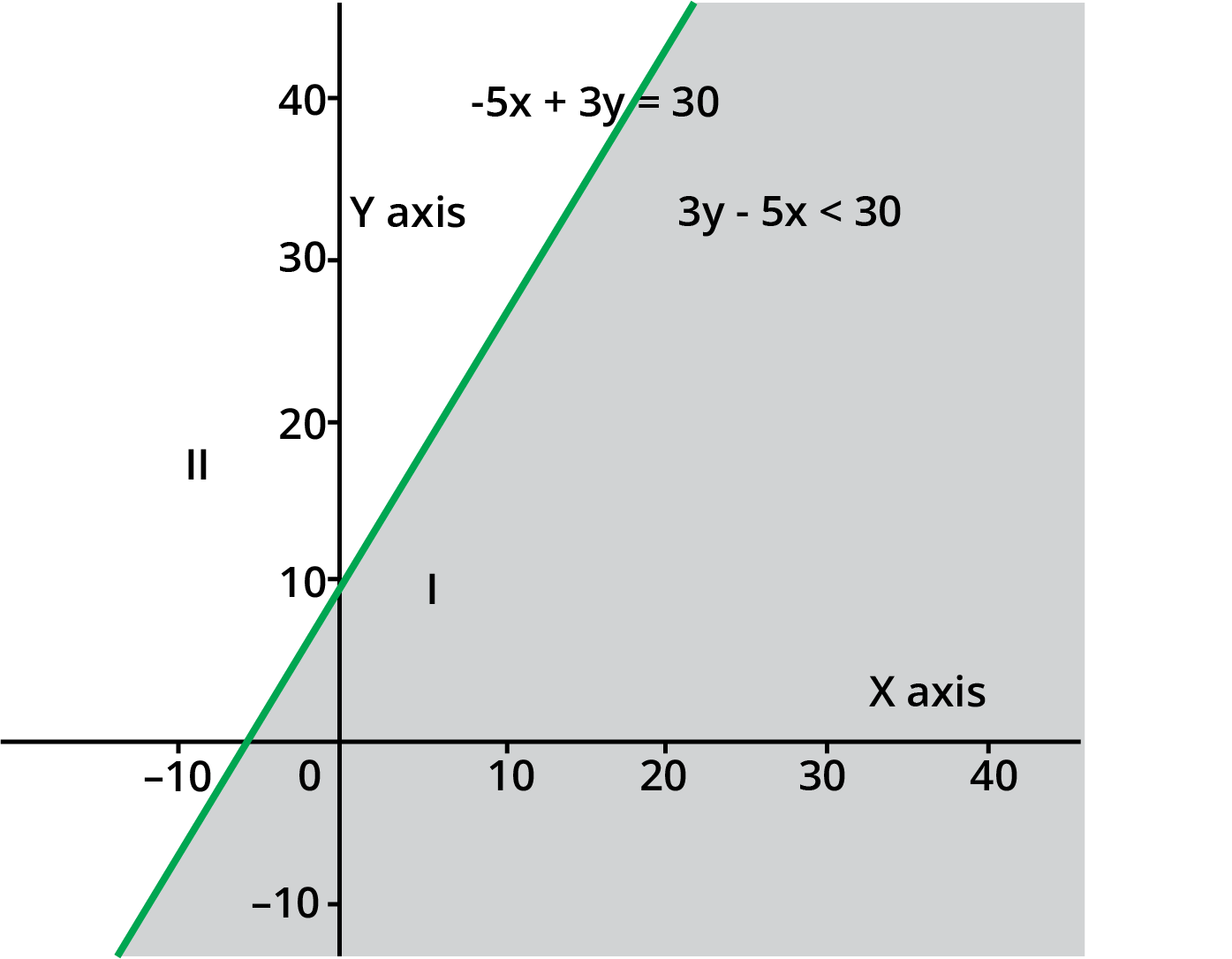9. Solve the given inequality graphically in two-dimensional plane: $\mathrm{y<-2}$

Ans: The graphical representation of the given plane $\text{y>-2}$ is given below.

The plane divides the $\text{x-y}$ plane into two planes $\text{I}$ and $\text{II}$.

Let us select a point which lies in any of the planes to check if the point satisfies the inequality or not.

Let us take $\left( 3,-3 \right)$, we get

$-3<-2$

Therefore we can say that the point satisfies the inequality.

Thus, we can say that the half plane $\text{II}$ does not satisfy the given inequality.

So, the graph is represented as below: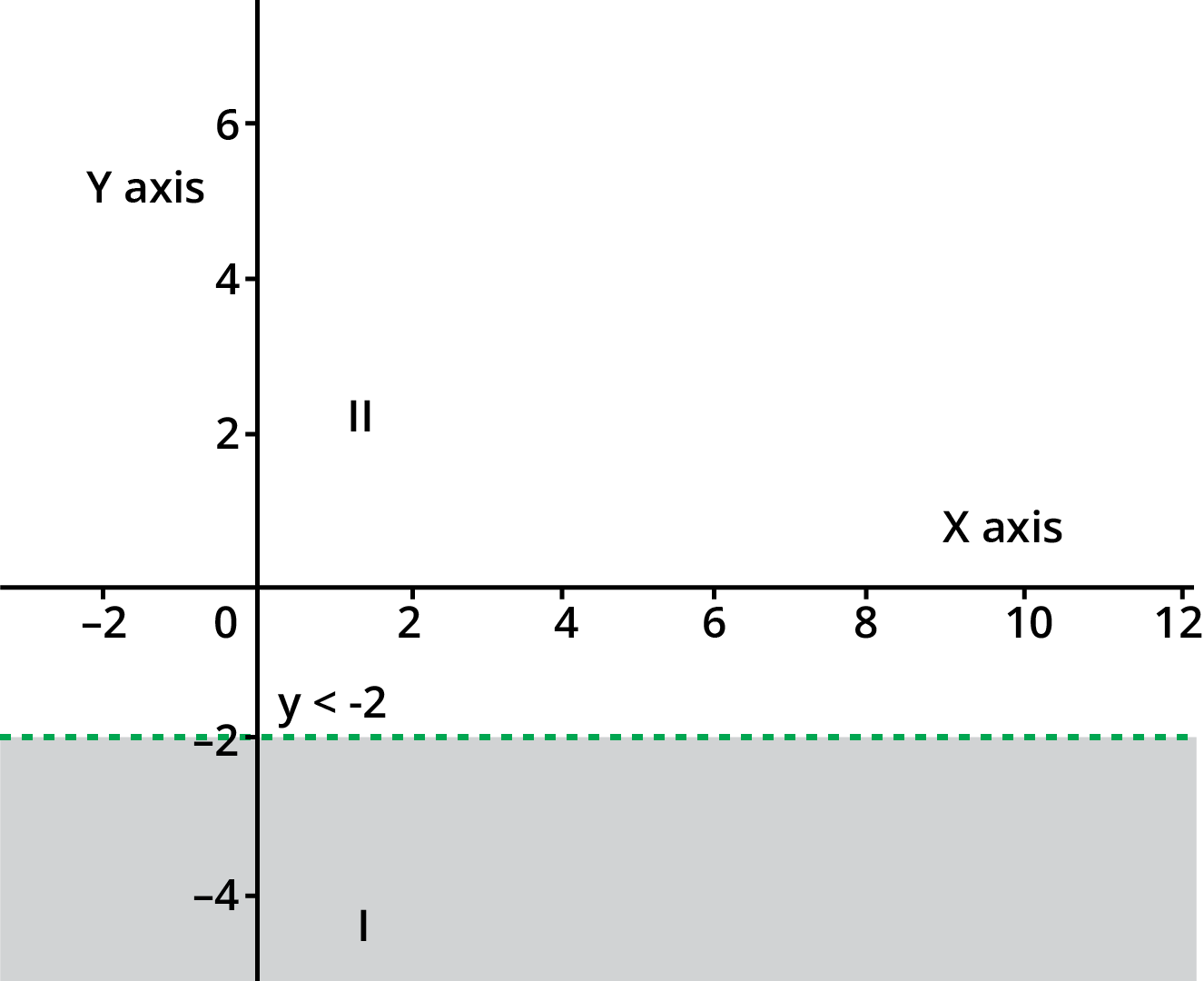10. Solve the given inequality graphically in two-dimensional plane: $\mathrm{x>-3}$

Ans: The graphical representation of the given plane $\text{x>-3}$ is given below.

The plane divides the $\text{x-y}$ plane into two planes $\text{I}$ and $\text{II}$.

Let us select a point which lies in any of the planes to check if the point satisfies the inequality or not.

Let us take $\left( -2,0 \right)$, we get $-2>-3$

Therefore we can say that the point satisfies the inequality.

Thus, we can say that the half plane $\text{II}$ does not satisfy the given inequality.

So, the graph is represented as below: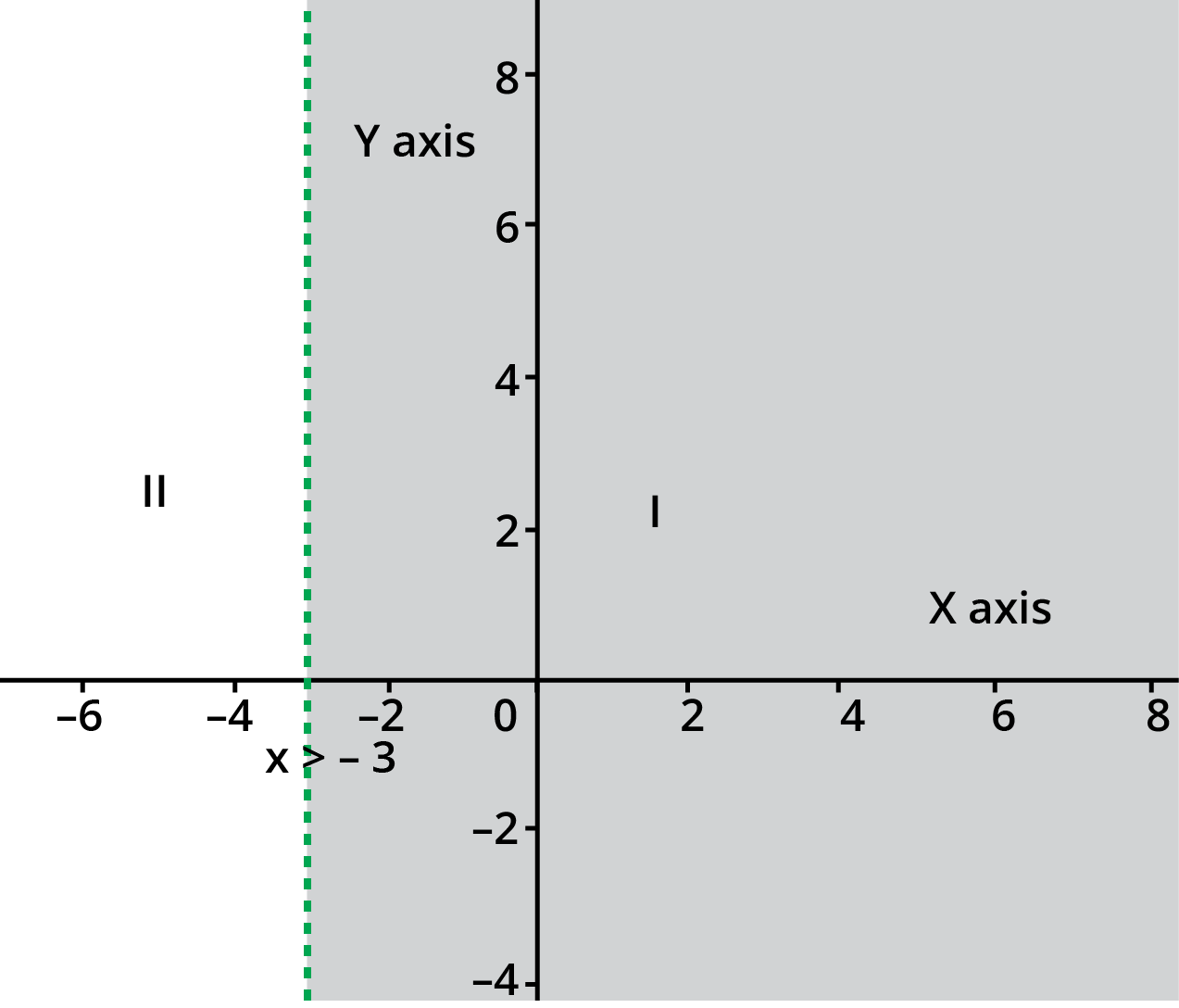## Class 11 Maths Chapter 6- Linear Inequalities

Chapter six of the mathematics subject for standard 11 states linear inequalities. It states the importance, the types and the day-to-day use of linear inequalities. The Chapter consists of all the important formulas that may come in handy while solving the questions. In addition to that, the revision notes for Class 11 Maths exercise 6.2 solution even consists of some easy tricks that will help the students in solving the questions faster. Though the tricks may not come in handy during school examinations, as the student has to show the whole procedure of solving the equations, the tricks will definitely help the students during the competitive exams, where they are required to solve the questions in a limited amount of time. The Chapter of linear inequalities is divided into several sections to help the students in knowing the different types of linear equations in a more detailed manner. The sections which are included in the NCERT Solutions for Class 11 Maths exercise 6.2 PDF and revision notes are stated below.

• Introduction to linear inequalities

• Examples of inequality in daily life

• Notations used in linear inequalities

• Types of linear inequalities

• Numerical inequalities

• Literal or variable inequalities

• Double inequalities

• Strict inequalities

• Slack inequalities

• Linear inequalities in one variable

• Linear inequalities in two variables

• Solution for a linear inequality in one variable solution and solution set

• Rules for inequality

• Examples for linear inequalities in one variable

• Graphical solution for linear inequalities in two variables

• Examples for solving linear inequalities in two variables in graphical representation

• Exercises

• Exercise 6.1

• Exercise 6.2

• Exercise 6.3

• Miscellaneous exercise

The Chapter of linear inequalities has been divided into several sections so that the students will be able to know the difference in the types of linear inequalities and would be able to solve the questions accordingly. The solutions for Class 11 Maths ch 6 ex 6.2 consists of bothe revision notes as well as the solutions to the questions given in the exercises. The solutions will help the students in knowing whether the process that they have followed for solving the question is correct or not. The Chapter even consists of several examples so that the students would get a brief idea of how to solve any similar type of questions. As it is clearly known that mathematics is a tricky subject and even one mistake, like changing of the sign, can lead to a whole different answers, the solutions are made with keeping in mind that the students do not get confused while solving the questions and also, the time taken for solving a question is not much.

### Introduction to Linear Inequalities

In this section of the PDF solutions for linear inequalities, the Chapter is introduced. In simple language, inequality is defined as a relation between two different values that holds true. A linear inequality is an inequality which consists of a linear function, where the equality sign is replaced with the symbol of inequality. The linear inequality function shows the data which is not equal in any form, either graphically or in a normal quadratic function. There are various types of linear inequalities like inequalities in one variable, two variables and many more.

The linear inequalities are studied to solve problems in the field of science. Most of the facts and theorems in the field of science cannot be solved by linear equations or by normal quadratic equations. There are many cases of probabilities and possibilities, where the actual solutions of the problems are not found. This is where linear inequalities come in use. The linear inequalities help the scientists and mathematicians in solving various theorems so that many complex problems can be solved easily. As linear inequalities, there are chances of getting more than one answer, in a finite set, they are mostly preferred while solving any hypothesis or complex problems.

### Examples of Inequality in Daily Life

The solutions PDF even consists of tricks and important key points, which would further help the students in revising the whole Chapter in a short span of time. It is known that mathematics can be difficult if it is not taught properly. To avoid this, Vedantu provides you with the best Maths professors who would teach you the Chapter of linear inequality and even provide you with some useful tricks which would help you in competitive exams, where time is short, and one is required to solve a large number of questions.

The section of applications or examples of inequality in daily life, states the purpose and how linear inequalities can help us in solving day to day problems in a better and easier way. There are various applications of linear inequalities which we use in our everyday life, without even knowing. One of the most common areas where linear inequalities find major application is linear programming and polyhedral. In the case of linear programming, the program or the problem requires a minimum and a maximum value for solving it. Apart from that, linear inequalities are used to solve problems regarding probabilities and possibilities, where both minimum and maximum values are required to find an approximate answer.

### Notations

The Notations section of the NCERT Solutions for Class 11 Maths exercise 6.2 speaks about the notations that are used while solving linear inequalities. There are various types of notations which are important and are used in linear inequalities. These notations are stated below

• The notation ‘<’ means less than. For example, a<b means that ‘a’ is less than ‘b.’

• The notation ‘>’ means greater than. For example, a>b means that ‘a’ is greater than ‘b’

• The notation of ‘≠’ means that both the variables or values are not equal. For example, a≠b means that the values in ‘a’ is not equal to that in ‘b’

• The notation ‘≤’ means that one value is less than or equal to the other value. For example, a≤b means that ‘a’ is less than or equal to ‘b’

• The notation ‘≥’ means that one value is greater than or equal to the other value in the given equation. For example, a≥b means that ‘a’ is greater than or equal to ‘b.’

The above-stated notations are some of the most important notations or symbols that are used in linear inequalities. These symbols help the person in knowing the values and the limits, according to which the solutions are formed.

### Types of Linear Inequalities

This section of the Class 11 Maths exercise 6.2 describes the various types of linear inequalities that are found in this particular field. Below are some of the common types of linear inequalities that we find in this field.

Numerical inequalities: Numerical inequalities are the most common type of inequality. As the name suggests, numerical inequalities state the inequality between numerals or real numbers. For example, 5<7, is a numerical inequality. We can even write the stated example as 5<(5+2), as the result would still be the same. Numerical inequalities define the basic or simple rules of mathematics which we learn from our primary classes.

Literal or variable inequalities: This type of inequality deals with the inequalities among variables and numbers or between two different variables. In this type of linear inequality, we find a relationship between numbers and variables or between two different variables. Here, there is no relation between the two numbers. For example, we can say that x>8 is a variable inequality or x>y is a variable inequality, but 8<9 is not a variable inequality. The last equation would come under numerical inequality, as it has numbers on both sides of the equation.

Double inequalities: Double inequalities are an upgraded version of variable inequalities. While in variable inequalities, we used the symbols for inequality on one side of the variable, here, we will use the symbols on both sides of the variable. For example, 5<x<9, which states that the variable ‘x’ has a relationship on both sides of the inequality symbol. Double inequalities are mainly used to show the limits for an equation which would further help the person to solve the question based on the limits provided.

Strict inequalities: Strict inequalities can be simply defined as the inequalities, which only use the symbols ‘<’ and ‘>’. In this type of inequality, you will not find the application of symbols like ‘≥’ or ‘≤’. The example of such inequalities is, x<4 or y>9 and many more.

Slack inequalities: While “strict inequalities” only use ‘<’ and ‘>’ symbols, the slack inequalities are the ones that use ‘≤’ and ‘≥’ symbols. Examples for slack inequalities are x≥8 or x≤3 and many more. We can say that slack inequalities are the opposite of strict inequalities.

Linear inequality in one variable: Linear inequality in one variable can be defined as the type of inequality which consists of a linear function with one variable. For example, x<8, can be defined as a linear inequality in one variable, as the equation comes under the category of linear inequality function due to the presence of an inequality symbol, and as it has one variable ‘x’ it can be said as a linear inequality with a single variable.

Linear inequalities in two variables: Linear inequalities in two variables include linear variables which consist of two variables in it. For example, x+6y>23, can be said to be a linear inequality function with two variables, as it has a linear inequality function due to the presence of an inequality symbol, and also it has two variables ‘x’ and ‘y’, and therefore it comes under the category of linear inequalities in two variables.

Quadratic inequalities: Quadratic inequalities, as the name suggests, involves a quadratic function, but instead of an equality symbol, the quadratic function has the symbol of inequality. For example, x2+2x≤78, comes under the category of quadratic inequalities, as the equation is a quadratic equation and has an inequality symbol in it.

### Solutions for a Linear Inequality in One Variable Solution and Solution Set

This particular section of the Class 11 Maths Chapter 6 exercise 6.2 PDF consists of the definitions which one may find in linear inequalities consisting of one variable. The solution, in a linear inequality consisting of one variable, is defined as the values of the variables, which makes the inequality as a true statement. For example, value 8 is a solution for linear inequality x>7.

Similarly, a solution set can be defined as the number of values that are possible to be the appropriate solutions for a particular linear inequality consisting of one variable. For example, the set of {1,2,3,4,5} is the solution set for the linear inequality x<6, as it consists of all the real numbers or values that can become the appropriate solution for the given linear inequality.

### Rules for Inequality

The “rules for inequality” section in the linear inequalities Chapter for standard 11, consists of the rules which should be followed while solving a linear inequality. These rules were formed by the experts in the field of mathematics, after lots of experiments and research. The rules should be strictly followed as it would help the students in solving the questions in the exercises. Below are the rules for solving linear inequality which will help you in solving the problems.

• The first rule states that equal numbers may be added to or subtracted from both sides of the inequality function, without affecting the notation of inequality in any manner. For example, for an inequality equation x<8, the equation can also be reframed as x+1<8+1, where the inequality notation has not been affected in any manner.

• The second rule for solving inequality equations is that both sides of an inequality function can either be multiplied or divided by the same positive numbers, without interfering with the notation of inequality in any manner. For example, x+y<8, can also be written as (x+y) *2< 8*2, where neither the equation is changed, nor has the notation for inequality has been affected.

• The third rule which should be kept in mind while solving linear inequalities is that, whenever both sides of the inequality equation are multiplied or divided by a negative number, the inequality sign will be reversed. For example, x+y<8, when multiplied by a negative number, becomes, (x+y)*(-2)> 8*(-2).

The above-stated rules will help you in solving simple linear inequalities in one variable easily. These rules will come in handy, and you can easily remember them too. The rules were devised by keeping in point that solving linear inequalities can be difficult and therefore, there must be some rules, which will turn the solving process of linear inequalities into a simpler way.

### Examples for Linear Inequalities in One Variable

The example section for linear inequalities in one variable consists of various questions and their solutions which will help the students in getting an idea about the type of questions they might face in this Chapter. The examples section for NCERT Solutions for Class 11 Maths exercises 6.2 consists of both short as well as long questions. While the short questions are easy and do not take much time to solve, the long questions follow a particular procedure and are time-consuming too. Though the solutions have been made as simple as possible so that the students will not face any difficulty while solving the problems. It is strictly advised to go through the examples before starting to solve the exercise problems, as they would help you in knowing the variety of questions and the different types of solutions that one has to adapt for solving a particular type of question.

### Graphical Solutions of Linear Inequalities in 2 Variables

While linear inequalities consisting of one variable can be solved using the rules that have been stated above, it is quite difficult to solve a linear inequality function consisting of two different variables. One of the most widely accepted processes for solving linear inequalities with two variables is by solving them through graphical representation. There are various steps which should be followed while solving linear inequalities with two variables by using the graphical representation process.

Firstly, a line is drawn through the Cartesian plane, which divides the plane into two halves after that follow the steps given below.

• The first step for solving linear inequalities with two variables is to replace the inequality symbol with an equality symbol and then plot the graph accordingly.

• After that, take any point in the graph and check whether it satisfies the linear inequality function or not. If the point satisfies the linear inequality function, then that region consists of the solution for the linear inequality.

In case of a slack inequality, use a solid line as the points in the solid line belong to a solid-state. In simple words, the slack inequality function consists of a finite number of values (with two limited values) and hence to represent them, we use a solid or bold line.

In case of a strict inequality, we use a dotted line. The reason as to why we use a dotted line for strict inequality function is that the strict inequality function consists of infinite number of values with only one limited values (as the starting value) and to represent them in a graph, we prefer a dotted line as it shows that the set is not crisp or finite.

### Examples for Solving Linear Inequalities With Two Variables Using a Graphical Representation

The examples section for linear inequalities with two variables by using graphical representation consists of various questions which would help the students in knowing the procedure for solving the questions for linear inequalities in two variables. It is not possible to solve the questions by just knowing the procedure, as one would definitely need examples to know how the procedure works. This particular example section consists of a variety of questions which would help you to know the steps and the appropriate methods which you can use for solving the questions. As stated earlier, it is important to go through the examples, as it will help you to know how you should solve the questions in order to achieve the correct solutions. The graphical representation process for solving linear inequalities with two variables is a bit difficult, and even after knowing the steps, it becomes a bit difficult to solve the exercise questions. Therefore, it is important to go through the examples and know the process of solving the questions. There are a variety of questions in the examples section along with tricks, which would help you in solving the questions easily.

### Exercises

The exercises section of Class 11 Maths Chapter 6 exercise 6.2 PDF consists of different questions which one might face during school examinations and even in competitive examinations. The exercises section will help you in knowing the different types of questions. As the solutions PDF consists of the solutions for the questions, you will get to know the process, methods and tricks that you can apply while solving the questions. The exercise section is divided into four different sections, which are stated below.

Exercise 6.1: The first section of the linear inequalities Chapter consists of questions based on simple linear inequalities, consisting of both short and long questions. The exercise consists of linear inequalities in single variables, indifferent manner, and different types so that the students will get to know the variety of questions and get acquainted with it. The solution part for this particular exercise not only consists of the classic long process which should be followed while solving the questions during board examinations; it even consists of tricks which will even help the students in solving the questions in a short span of time during competitive exams.

Exercise 6.2: The linear inequalities Class 11 exercise 6.2 consists of questions based on linear inequalities with two variables. As the solving methods for linear inequalities with two variables is a bit different and are difficult too; therefore, it is important to solve the questions from this exercise section. The questions found in Class 11 Maths exercise 6.2 is similar to the examples section for linear inequalities with two variables, it would be easy for students to solve the questions, once he/she has gone through the examples properly. The solutions part even consists of the graphs for each question, therefore making it easier for the students to solve the questions.

Exercise 6.3: The exercise 6.3 of linear inequalities Chapter for standard 11, consists of a mixture of questions from both linear inequalities consisting of one variable and linear inequalities consisting of two variables. As it consists of a mixture of both types of linear inequalities, it becomes easier for the students to revise the Chapter and recall the methods and steps which one should follow while solving the questions. This particular exercise was designed by keeping in mind that the students would get a mixture of questions in board exams and competitive exams, and therefore it would be better to help them in revising the whole Chapter. The solutions consist of all the rules for both linear inequalities with one and two variables, and hence it would become easier for you to revise the Chapter in a short span of time. In case you need to revise the Chapter before exams, this exercise is a must.

Miscellaneous exercise: This particular exercise not only consists of a mixture of a variety of questions from the Chapter but also consists of questions which had appeared in previous board examinations as well as competitive examinations. In case you want to know the type of questions that appear on the board as well as competitive examinations, this particular exercise will help you in getting that. The solutions are quite easy so that the students do not have to go through a long process, and do not have to spend more time solving them.